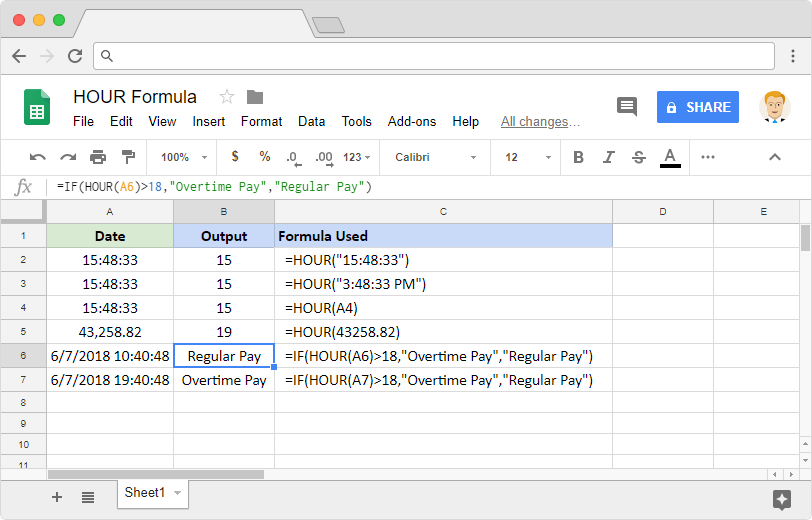# How to use the HOUR formula in Google SheetsAt times we may need to pick the hour from a time value. Possibly to use it with another formula that calculates an overtime paycheck. Manually, we can quickly identify the hour of the day by looking at the time. However, the machine cannot directly deduce it without additional help. Because, just like the date, time is also is a numeric value on the spreadsheets application. For example, the underlying numeric value for “19:40:48” is 0.82. So how do we extract the hour of the day from this numeric value? The HOUR formula in Google Sheets can help us do that.

### Syntax

HOUR(time)

time – is the time which we need to extract the hour component from. This input has to be a date/time type data. We can ensure that either by using formulas that return date/time objects. Or we may also use references to the cells that have dates or numbers.

### Usage: HOUR formula in Google Sheets

It is very simple and straightforward and to use this formula because it takes in just one compulsory parameter. We just reviewed the syntax, now it is time to concrete our understanding with the help of a few practical examples. Please see the image below.You’ll see that we have tried multiple possible ways in which we can pass the value for the input parameter. In the first example, we passed a valid time string. So, the formula has returned the value 15 as expected. The second example proves that the formula can also take in AM/PM time formatting, yet returns the hour as 15. Because it is the same input as in the first example.

The third example demonstrates the capability of the formula to accept the references to cells that have dates/times within them. The fourth example worked even when there’s a number on the cell. For those of us who are wondering what the rational number 43,258.82 corresponds to, it stands for “7-Jun-2017 7:40:48 PM” in Google Sheets. Hence the formula returned the value 19 for output.

Now, let us move on to more practical examples on the rows 6 and 7. Here we are trying to deduce whether an hour qualifies for the overtime pay or not. And the composite formula we built does it seamlessly.

To extract the day of the month from a given date, check out the following blog post on how to use the DAY formula in Google Sheets.